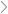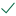Questions & AnswersC++ Programming// Interface to the Wall ADT // !!! DO NOT MODIFY THIS FILE !!!...

QuestionAnswered step-by-step

# // Interface to the Wall ADT // !!! DO NOT MODIFY THIS FILE !!!...

// Interface to the Wall ADT

// !!! DO NOT MODIFY THIS FILE !!!

#ifndef WALL_H
#define WALL_H

typedef struct wall *Wall;

// Rock colours
#define NUM_COLOURS 4

// enums are used to create groups of related constants. It is like
// creating multiple #defines, except you can also provide a type name.
typedef enum {
GREEN = 0,
TEAL  = 1,
PINK  = 2,
RED   = 3,
} Colour;

// Terminal output colours
#define GRN "\x1B[32m"
#define CYN "\x1B[96m"
#define MAG "\x1B[95m"
#define RD  "\x1B[91m"
#define RESET "\x1B[0m"

struct rock {
int row;
int col;
Colour colour;
};

// Implementation of the Wall ADT

#include <assert.h>
#include <math.h>
#include <stdbool.h>
#include <stdio.h>
#include <stdlib.h>

#include "Wall.h"

struct wall {
// TODO
int height;
int width;
struct rock **rocks;
int num_rocks;
int rows;
int cols;
struct rock ***grid;
};

static int compareRocks(const void *ptr1, const void *ptr2);

/**
* Creates a new blank wall with the given dimensions
*/
Wall WallNew(int height, int width) {
// TODO
Wall w = malloc(sizeof(*w));
w->height = height;
w->width = width;
w->num_rocks = 0;
w->rocks = malloc(height * sizeof(struct rock *));

for (int i = 0; i < height; i++) {
w->rocks[i] = calloc(width, sizeof(struct rock));
}

//Initialising the grid
w->rows = height + 2 ;
w->cols = width + 2 ;
w->grid = calloc(w->rows, sizeof(struct rock **));
for (int i = 0; i < w->rows; i++) {
w->grid[i] = calloc(w->cols, sizeof(struct rock *));
}
return w;

}

/**
* Frees all memory allocated to the wall
*/
void WallFree(Wall w) {
// TODO
for (int i = 0; i < w->height; i++) {
free(w->rocks[i]);
}
free(w->rocks);
for (int i = 0; i < w->rows; i++) {
free(w->grid[i]);
}
free(w->grid);
free(w);
}

/**
* Returns the height of the wall
*/
int WallHeight(Wall w) {
// TODO
return w->height;
}

/**
* Returns the width of the wall
*/
int WallWidth(Wall w) {
// TODO
return w->width;
}

/**
* Adds a rock to the wall
* If there is already a rock at the given coordinates, replaces it
*/
void WallAddRock(Wall w, struct rock rock) {
// TODO
if (rock.row >= 1 && rock.row <= w->height && rock.col >= 1 && rock.col <= w->width) {
w->rocks[rock.row - 1][rock.col - 1] = rock;
// Update the grid
w->grid[rock.row][rock.col] = &(w->rocks[rock.row - 1][rock.col - 1]);
w->num_rocks++;
} else {
fprintf(stderr, "Invalid rock coordinates (%d, %d)\n", rock.row, rock.col);
}
}

/**
* Returns the number of rocks on the wall
*/
int WallNumRocks(Wall w) {
// TODO
return w->num_rocks;
}

/**
* Stores all rocks on the wall in the given `rocks` array and returns
* the number of rocks stored. Assumes that the array is at least as
* large as the number of rocks on the wall.
*/
int WallGetAllRocks(Wall w, struct rock rocks[]) {
// TODO
int num_rocks = 0;
for (int row = 1; row <= w->height; row++) {
for (int col = 1; col <= w->width; col++) {
if (w->grid[row][col] != NULL) {
rocks[num_rocks] = *(w->grid[row][col]);
num_rocks++;
}
}
}
// Sort the rocks by row, then by column
qsort(rocks, num_rocks, sizeof(struct rock), compareRocks);
return num_rocks;

}

/**
* Stores all rocks that are within a distance of `dist` from the given
* coordinates in the given `rocks` array and returns the number of rocks
* stored. Assumes that the array is at least as large as the number of
* rocks on the wall.
*/
int WallGetRocksInRange(Wall w, int row, int col, int dist,
struct rock rocks[])
{
// TODO
int num_rocks = 0;
for (int r = 1; r <= w->height; r++) {
for (int c = 1; c <= w->width; c++) {
// Calculate the distance between the two points using the Pythagorean theorem
int dx = abs(c - col);
int dy = abs(r - row);
int distance = sqrt(dx * dx + dy * dy);
// Add the rock to the array if it is within the given distance and not NULL
if (distance <= dist && w->grid[r][c] != NULL) {
rocks[num_rocks] = *(w->grid[r][c]);
num_rocks++;
}
}
}
return num_rocks;
}

/**
* Stores all rocks with the colour `colour` that are within a distance
* of `dist` from the given coordinates in the given `rocks` array and
* returns the number of rocks stored. Assumes that the array is at
* least as large as the number of rocks on the wall.
*/
int WallGetColouredRocksInRange(Wall w, int row, int col, int dist,
Colour colour, struct rock rocks[])
{
// TODO
int num_rocks = 0;
for (int r = 1; r <= w->height; r++) {
for (int c = 1; c <= w->width; c++) {
// Calculate the distance between the two points using the Pythagorean theorem
int dx = abs(c - col);
int dy = abs(r - row);
int distance = sqrt(dx * dx + dy * dy);
// Add the rock to the array if it is within the given distance, has the given color, and not NULL
if (distance <= dist && w->grid[r][c] != NULL && w->grid[r][c]->colour == colour) {
rocks[num_rocks] = *(w->grid[r][c]);
num_rocks++;
}
}
}
return num_rocks;
}

////////////////////////////////////////////////////////////////////////

/**
* Prints the wall out in a nice format
* NOTE: DO NOT MODIFY THIS FUNCTION! This function will work once
*       WallGetAllRocks and all the functions above it work.
*/
void WallPrint(Wall w) {
int height = WallHeight(w);
int width = WallWidth(w);
int numRocks = WallNumRocks(w);
struct rock *rocks = malloc(numRocks * sizeof(struct rock));
WallGetAllRocks(w, rocks);
qsort(rocks, numRocks, sizeof(struct rock), compareRocks);

int i = 0;
for (int y = height; y >= 0; y--) {
for (int x = 0; x <= width; x++) {
if ((y == 0 || y == height) && (x == 0 || x % 5 == 0)) {
printf("+ ");
} else if ((x == 0 || x == width) && (y == 0 || y % 5 == 0)) {
printf("+ ");
} else if (y == 0 || y == height) {
printf("- ");
} else if (x == 0 || x == width) {
printf("| ");
} else if (i < numRocks && y == rocks[i].row && x == rocks[i].col) {
char *color;
switch (rocks[i].colour) {
case GREEN: color = "\x1B[32m"; break;
case TEAL:  color = "\x1B[96m"; break;
case PINK:  color = "\x1B[95m"; break;
case RED:   color = "\x1B[91m"; break;
default:    color = "\x1B[0m";  break;
}
printf("%so %s", color, RESET);
i++;
} else {
printf("\u00B7 ");
}
}
printf("\n");
}

free(rocks);
}

static int compareRocks(const void *ptr1, const void *ptr2) {
struct rock *r1 = (struct rock *)ptr1;
struct rock *r2 = (struct rock *)ptr2;
if (r1->row != r2->row) {
return r2->row - r1->row;
} else {
return r1->col - r2->col;
}
}

Given the code above which is the interface and the implementation of the WALL ADT. I have completed all the functions for it and now i need help to write up the code for the functions below using the functions from the WALL ADT, which is why i have included the code above.

// Interface to boulder climbing algorithms

// !!! DO NOT MODIFY THIS FILE !!!

#ifndef CLIMBER_H
#define CLIMBER_H

#include "Wall.h"

struct path {
int numRocks;
struct rock *rocks;
};

// Implementation of boulder climbing algorithms

#include <assert.h>
#include <limits.h>
#include <math.h>
#include <stdbool.h>
#include <stdio.h>
#include <stdlib.h>

#include "climber.h"
#include "Wall.h"

struct path findShortestPath(Wall w, int reach, Colour colour) {
struct path p = {0, NULL};
return p;
}

struct path findMinEnergyPath(Wall w, int reach, int energyCosts[NUM_COLOURS]) {
struct path p = {0, NULL};
return p;
}

struct path findMinTurnsPath(Wall w, int reach, int energyCosts[NUM_COLOURS],
int maxEnergy) {
struct path p = {0, NULL};
return p;
}

I need help writing the code for the above functions using the functions in the WALL ADT. For instance instead of using w->height, i need to use WallHeight(w). I cant use the stab notation. So none of the following are meant to be written in my code wall->height, wall->width, wall->grid[][]. Hope this helps.

Here is some example code, except this doesnt work this is just for a rough idea. This doesnt work because the function bool canReach has no x or y members in the struct rock. So how do i change this?Solved by verified expert
``sectetur adipiscing elit. Nam lacinia pulvinar tortor nec facilisis. Pellentesque dapibus efficitur laoreet. Nam risus ante, dapibus a molestie consequat, ultrices ac magna. Fusce dui lectus, congue vel laoreet ac, dictum vitae odio. Donec aliquet. Lorem i``10,000 step-by-step explanations

``sectetur adipiscing elit. Nam lacinia pulvinar tortor nec facilisis. Pellentesque dapibus efficitur laoreet. Nam risus ante, dapibus a molestie consequat, ultrices ac magna. Fusce dui lectus, congue vel laoreet ac, dictum vitae odio. Donec aliquet. Lorem ipsum dolor sit amet, consectetur adipiscing elit. Nam lacinia pulvinar tortor nec facilisis. Pellentesque dapibus efficitur laoreet. Nam risus ante, dapibus a molestie consequat, ultrices ac magna. Fusce dui lectus, congue vel laoreet ac, dictum vitae odio. Donec aliquet. Lorem ipsum dolor sit amet, consectetur adipiscing elit. Nam lacinia pulvinar tortor nec facilisis. Pellentesque dapibus efficitur laoreet. Nam risus ante, dapibus a molestie consequat, ultrices ac magna. Fusce dui lectus, congue vel laoreet ac, dictum vitae odio. Donec aliquet. Lorem ipsum dolor sit amet, consectetur adipiscing elit. Nam lacinia pulvinar tortor nec facilisis. Pellentesque dapibus efficitur laoreet. Nam risus ante, dapibus a molestie consequat, ultrices ac magna. Fusce dui lectu``